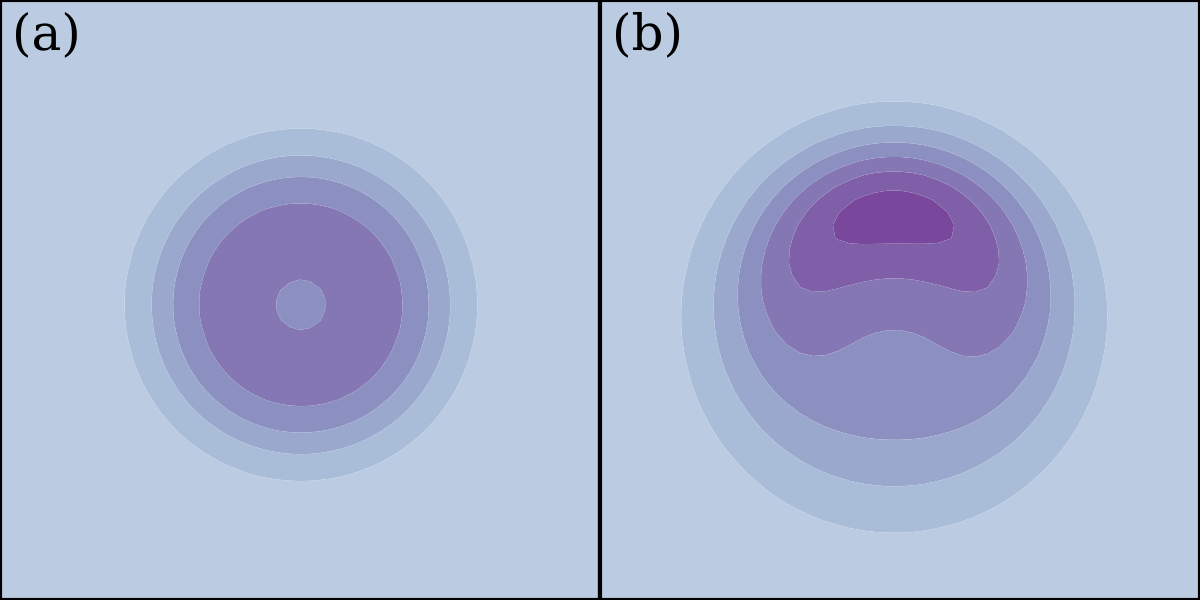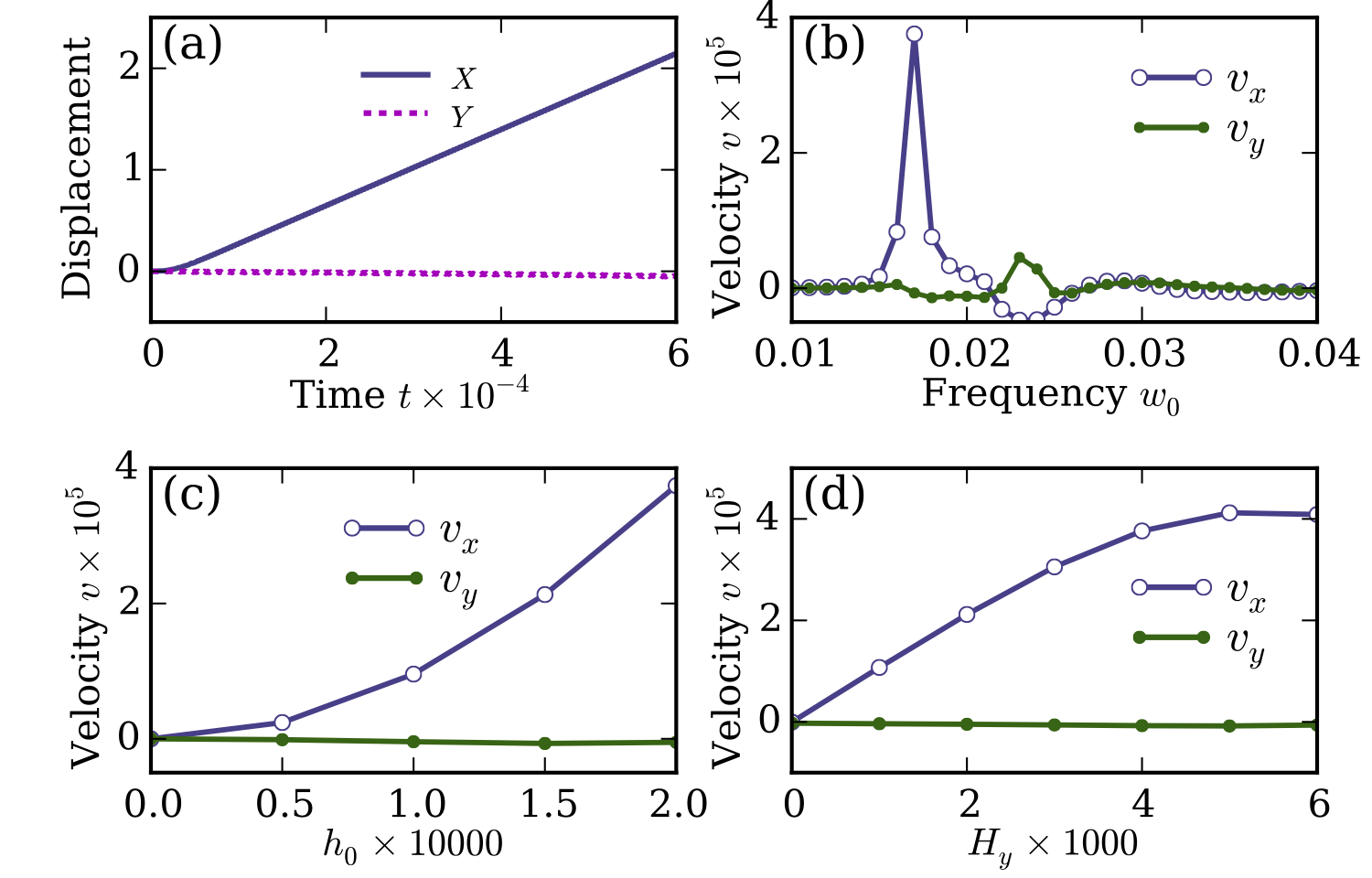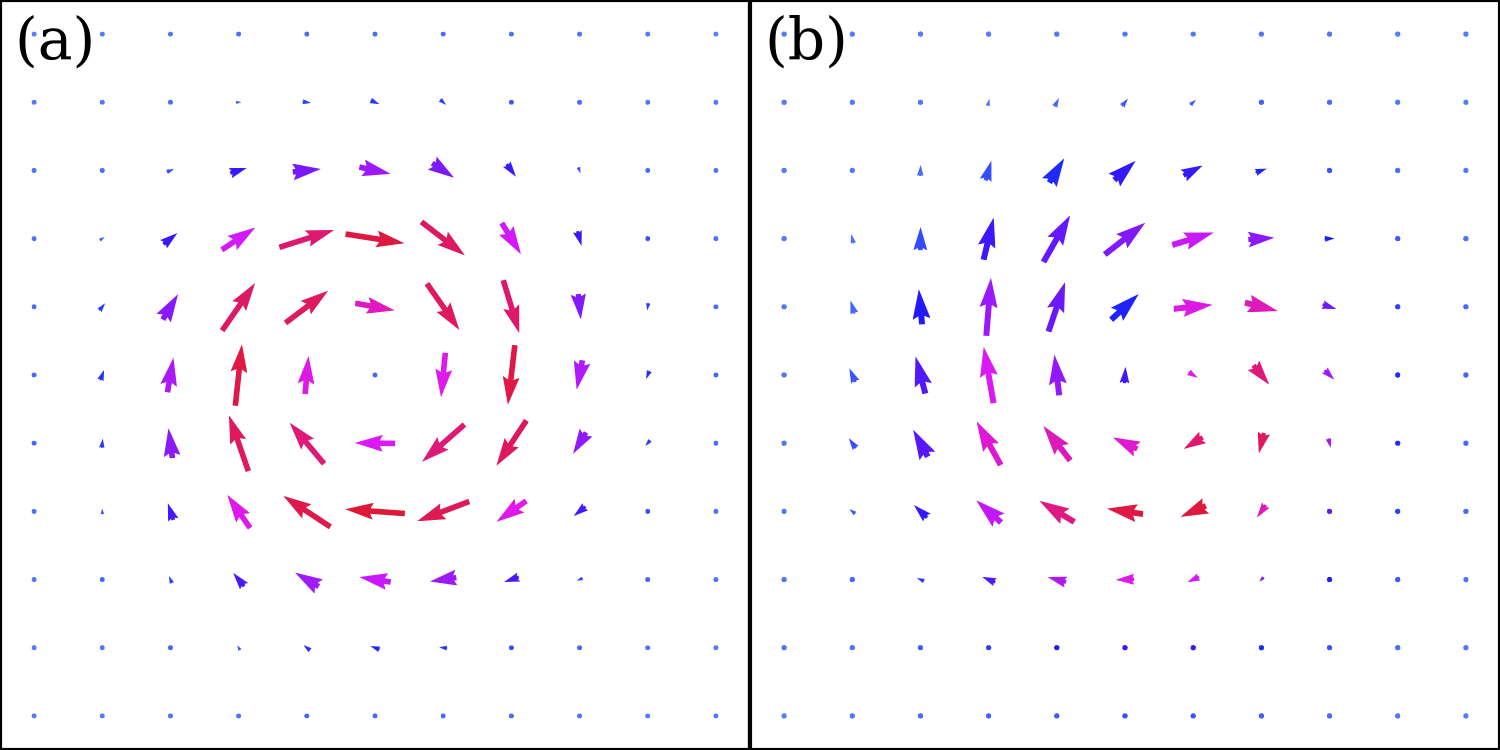# Driving magnetic skyrmions using microwaves

Summary of our recent paper on driving magnetic skyrmions with microwave fields

Weiwei Wang, Marijan Beg, Bin Zhang, Wolfgang Kuch, and Hans Fangohr
Driving magnetic skyrmions with microwave fields
Physical Review B 92, 020403(R) (2015)
(Online: journal arXiv.org)

## Context: Magnetic skyrmions

A very brief introduction to magnetic skyrmions can be found here.

In short, skyrmions are topologically stable magnetization textures with particle-like properties. They can be very small and also easily manipulated using various different methods.

### Known methods to move skyrmions

• spin-polarized current
• magnetic or electric field gradients
• magnons

## In this study

We demonstrate that a skyrmion can be moved by application of a periodic external magnetic field (called a 'microwave field' below). However, a key requirement is that the symmetry of the skyrmion configuration must be broken for this field driven process to be effective.

### The simulation setup

• The simulated geometry is a two-dimensional square lattice.
• The system's Hamiltonian contains symmetric exchange, (bulk) Dzyaloshinskii-Moriya, and Zeeman energy contributions.
• A set of applied external magnetic fields are:
• Static external magnetic field in the z-direction to stabilise skyrmion configuration.
• Adjustable in-plane external magnetic field to break the symmetry of a skyrmion configuration.
• Driving periodic microwave field in z-direction.

### Axially symmetric vs axially asymmetric skyrmions(a) The symmetric topological charge density distribution of a single magnetic skyrmion. (b) The corresponding topological charge density for the skyrmion in the presence of a small in-plane field.

## Simulation results(a) The skyrmion moves in the presence of in-plane field and microwave fields. (b) The skyrmion velocity dependence on the microwave frequency. This demonstrates that a single skyrmion has the maximum velocity when the microwave frequency corresponds to the skyrmion's breathing mode resonance frequency. (c) The dependence of velocity on the microwave's amplitude, showing that the skyrmion's velocity can be increased by changing the microwave's amplitude. (d) The dependence of velocity on the applied in-plane external magnetic field. The more strongly the symmetry is broken, the higher the velocity.This figure plots the total spatial force density defined in equation (6) in the paper for (a) an axially symmetric skyrmion (in absence of an in-plane magnetic field) and (b) an axially asymmetric skyrmion (in presence of an in-plane magnetic field). We can see in (a) that the force density is symmetric and thus the net force on a skyrmion is zero. However, as shown in figure (b), the force distribution becomes asymmetric, resulting in the skyrmion motion due to the nonzero net force.

## Summary

• We study the manipulation a skyrmion using a microwave field.
• Our results demonstrate that skyrmions can be effectively moved using microwave fields if the axial symmetry of the skyrmions is broken. The skyrmion's symmetry can be broken using a static in-plane external magnetic field.

## Future work

Magnetic skyrmion is an active field of research - if you are interested to pursue a PhD in computational modelling of such systems, please get in touch.

## Full text access and paper details

Weiwei Wang, Marijan Beg, Bin Zhang, Wolfgang Kuch, and Hans Fangohr
Driving magnetic skyrmions with microwave fields
Physical Review B 92, 020403(R) (2015)

PDFs are available from: